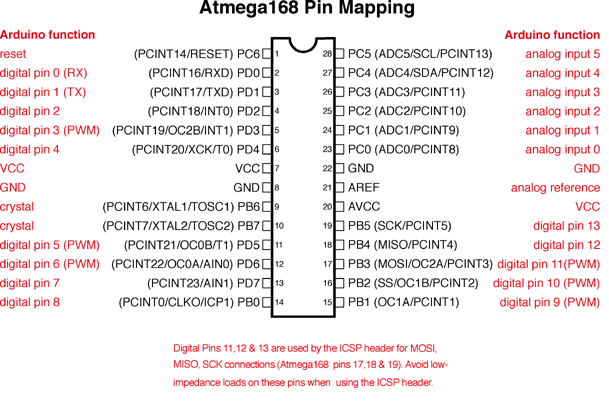# ATmega328PB Arduino Pin Mappings

I’m using a breadboarded ATmega328PB with platformio. I’m able to address pins in native code no problem, but using pin labels such as PD5, PD6 etc in Arduino code has no effect, i.e. the code compiles correctly but the expected outputs do not occur. PD7, strangely, does work. Can anyone help? This is driving me even more mad than I was to start with.

This is platformio.ini (including irrelevant bits for completeness):
[env:atmega328pb]
platform = atmelavr
board = atmega328pb
framework = arduino
board_build.mcu=atmega328pb
board_build.f_cpu=8000000L

This is the native code that works:
#include <avr/io.h>
#include <util/delay.h>

``````#define INTERVAL 100

void lights();

int main(void) {
DDRB = 1 << 1;  // make the RED LED pin an output for PORTD5
DDRC = 1 << 1;  // make the YELLOW LED pin an output for PORTD6
DDRD = 1 << 7;  // make the GREEN LED pin an output for PORTD7

while (1)
{
lights();
_delay_ms(INTERVAL);
lights();
_delay_ms(INTERVAL);
}
}

void lights() {
PORTB ^= 1 << 1;
PORTC ^= 1 << 1;
PORTD ^= 1 << 7;
}
``````

This is the Arduino code that doesn’t work (except PD7):
#include <arduino.h>

``````#define GREENLED PD7
#define REDLED PD6
#define YELLOWLED PD5

#define INTERVAL 100

void lights();

void setup() {
pinMode(GREENLED, OUTPUT);
pinMode(REDLED, OUTPUT);
pinMode(YELLOWLED, OUTPUT);
}

void loop() {
lights();
delay(INTERVAL);
lights();
delay(INTERVAL);
}

void lights() {
}``````

The pin mapping isSo here PD7 = D7, PB0 = D8. The `pinMode` functions etc. take the number of the digital pin, e.g. “7” for PD7, and “8” for PB0.

Let’s look at how this expression is expanded:

``````digitalWrite(PD7, 1);
``````

`Arduino.h` includes `avr/portpins.h` in which it says

``````#if defined(PD5) && !defined(PORTD5)
#  define PORTD5 PD5
#elif defined(PORTD5) && !defined(PD5)
#  define PD5 PORTD5
#endif
#if defined(PD6) && !defined(PORTD6)
#  define PORTD6 PD6
#elif defined(PORTD6) && !defined(PD6)
#  define PD6 PORTD6
#endif
#if defined(PD7) && !defined(PORTD7)
#  define PORTD7 PD7
#elif defined(PORTD7) && !defined(PD7)
#  define PD7 PORTD7
#endif
``````

Which leads to `avr/iom328p.h`:

``````#define PORTD0 0
#define PORTD1 1
#define PORTD2 2
#define PORTD3 3
#define PORTD4 4
#define PORTD5 5
#define PORTD6 6
#define PORTD7 7
``````

So the expression expands to

``````//digitalWrite(PD7, 1);
digitalWrite(7, 1);
``````

which works because PD7 is actually digital pin 7.

However, “PB5” would be expanded to the expression “5”, which is wrong. Digital pin 5 corresponds to PD5 on the chip.

``````#  define PB5 PORTB5
#define PORTB5 5
``````

So we see that the macros `PD5` and `PB5` both equal to 5. Thus they’re impossible to distuingish alone.

In short: Arduino APIs like pinMode, `digitalWrite`, `digitalRead`, take digital pin numbers as assigned in the pin mapping, not macros like `PD5` et cetera, Some of them happen to coincide with the correct value, however.

1 Like

Thank you Max for a very clear explanation of why PD5 and PD6 won’t work in the Arduino framework. It’s just like the old Milennium Bug - a simplification which leads to a lack of capacity.

So the next question, inevitably, is this… is there an Arduino framework way of addressing these pins for the digitalRead/digitalWrite functions rather than using their port/bit designations?

This is more for code consistency than anything. I’m trying to come up with a “best compromise” set of guidelines for a mixed skill dev team.

Thanks,
Jon

Yes, just use the pin numbers. There aren’t additional definitions like “D0 = 0, D1 = 1, …, D13 = 13” (write them yourself if you must), but there’s `const int` definitions for “A0” to “A5” if you need to address the analog functions in a `pinMode`, `digitalRead/Write` call (PC0 to PC5). I’d just make everyone use Arduino “digital pin 0…13, A0…A5” pin numbering consistently. When using low-level code, comment extensively.

If you do need to make direct port manipulations (for speed), consider either writing the easily understandable code using macros (`Arduino.h` has lots of them defined)

``````#define bit(b) (1UL << (b))
#define bitRead(value, bit) (((value) >> (bit)) & 0x01)
#define bitSet(value, bit) ((value) |= (1UL << (bit)))
#define bitClear(value, bit) ((value) &= ~(1UL << (bit)))
#define bitWrite(value, bit, bitvalue) (bitvalue ? bitSet(value, bit) : bitClear(value, bit))

// Get the bit location within the hardware port of the given virtual pin.
// This comes from the pins_*.c file for the active board configuration.
//
// These perform slightly better as macros compared to inline functions
//
#define digitalPinToPort(P) ( pgm_read_byte( digital_pin_to_port_PGM + (P) ) )
#define digitalPinToTimer(P) ( pgm_read_byte( digital_pin_to_timer_PGM + (P) ) )
#define portOutputRegister(P) ( (volatile uint8_t *)( pgm_read_word( port_to_output_PGM + (P))) )
#define portInputRegister(P) ( (volatile uint8_t *)( pgm_read_word( port_to_input_PGM + (P))) )
#define portModeRegister(P) ( (volatile uint8_t *)( pgm_read_word( port_to_mode_PGM + (P))) )
``````
``````//bad: (readability)
PORTB ^= 1 << 1;
//better:
// flip output pin PB1 (corresponds to D9).
//since PB = 1 we flip the 2nd bit from the right. (1 << 1)
PORTB ^= bit(PB1);
//or, slower

PORTD &= ~(1 << 5);
//better:
//set pin PD5 = D5 to 0.
bitClear(PORTD, PD5);
//or just (slower)
digitalWrite(5, LOW);
``````

There are also functions which convert Arduino pin numbers to bit masks or port pointers. Take a look at
Internal Structure of Arduino Software (`digitalPinToBitMask()`, …). This is what the Arduino framework internally uses inside the `pinMode`, `digitalWrite` etc functions to do to the pin re-mapping.

Or use the digitalWriteFast library which compile-time evaluates to the correct expressions.

• digitalWriteFast(pinNum, state) (sets or clears pin/port faster)
• pinModeFast(pinNum, mode) (sets pin/port as input or output faster)